Article Outline

函数

• 它的输入是怎么构成的（都有哪些参数？如何指定？）；
• 以及它的输出是什么（返回值究竟是什么？）……

NOTE

https://docs.python.org/3/library/functions.html

示例 print()

基本的使用方法

print() 是初学者最常遇到的函数 —— 姑且不说是不是最常用到的。

print('line 1st')
print('line 2nd')
print()
print('line 4th')
line 1st
line 2nd

line 4th

print('Hello,', 'jack', 'mike', '...', 'and all you guys!')
Hello, jack mike ... and all you guys!

name = 'Ann'
age = '22'
print(f'{name} is {age} years old.')
Ann is 22 years old.

name = 'Ann'
age = '22'
a = f'{name} is {age} years old.'
print(a)
'Ann is 22 years old.'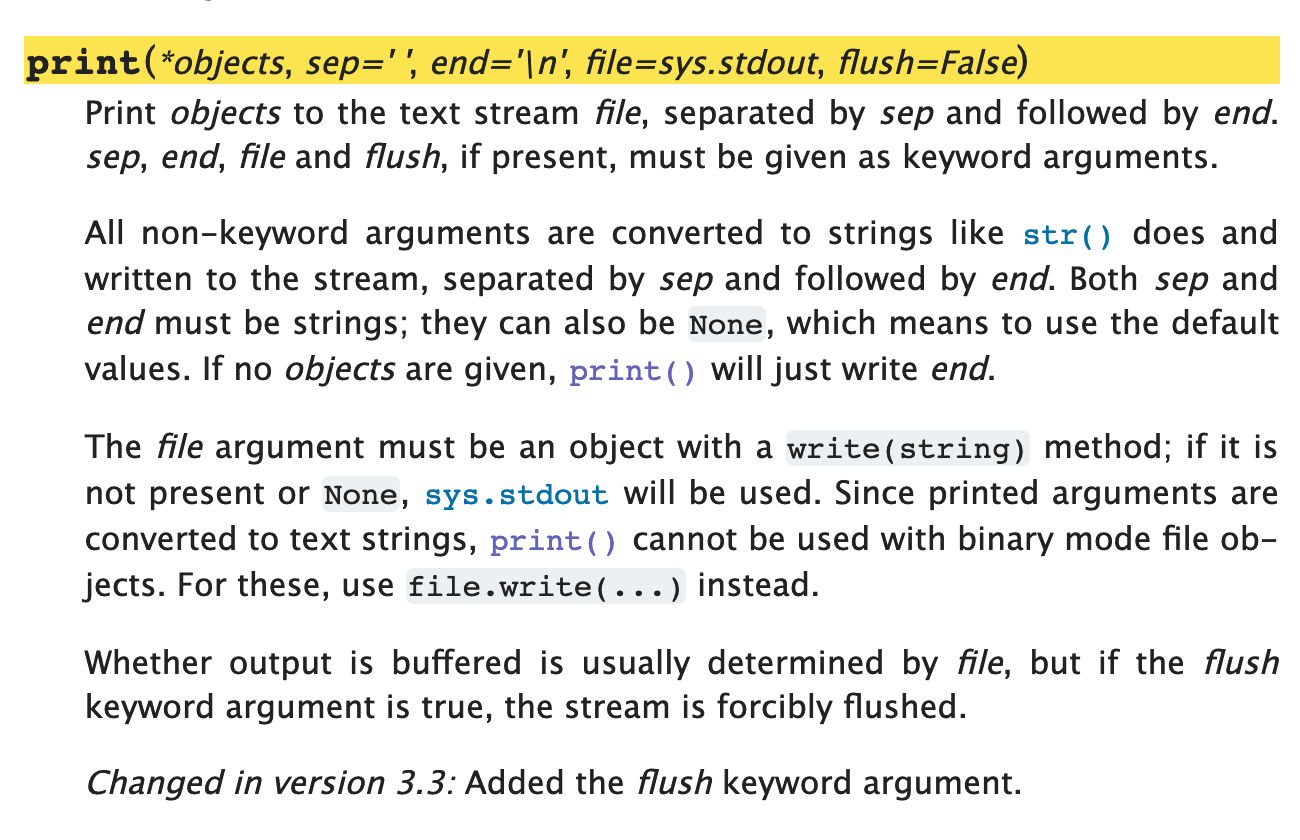print(*object, sep=' ', end='\n', file=sys.stdout, flush=False) 20

• sep=' '：接收多个参数之后，输出时，分隔符号默认为空格，' '
• end='\n'：输出行的末尾默认是换行符号 '\n'
• file=sys.stdout：默认的输出对象是 sys.stdout（即，用户正在使用的屏幕）……

import sys                                  # 如果没有这一行，代码会报错

print('Hello', 'world!')                    # 下一行的输出和这一行相同
print('Hello', 'world!', sep=' ', end='\n', file=sys.stdout, flush=False)
print('Hello', 'world!', sep='-', end='\t')
print('Hello', 'world!', sep='~')           # 上一行的末尾是 \t，所以，这一行并没有换行显示
print('Hello', 'world!', sep='\n')          # 参数之间用换行 \n 分隔
Hello world!
Hello world!
Hello-world!    Hello~world!
Hello
world!

print() 这个函数的返回值是 None —— 注意，它向屏幕输出的内容，与 print() 这个函数的返回值不是一回事。

print(print(1))
1
None

关键字参数

• 位置参数（Positional Arguments，在官方文档里常被缩写为 arg
• 关键字参数（Keyword Arguments，在官方文档里常被缩写为 kwarg

sorted(iterable, *, key=None, reverse=False)

a = sorted('abdc')
b = sorted('abdc', reverse=True)
print(a)
print(b)
['a', 'b', 'c', 'd']
['d', 'c', 'b', 'a']

位置参数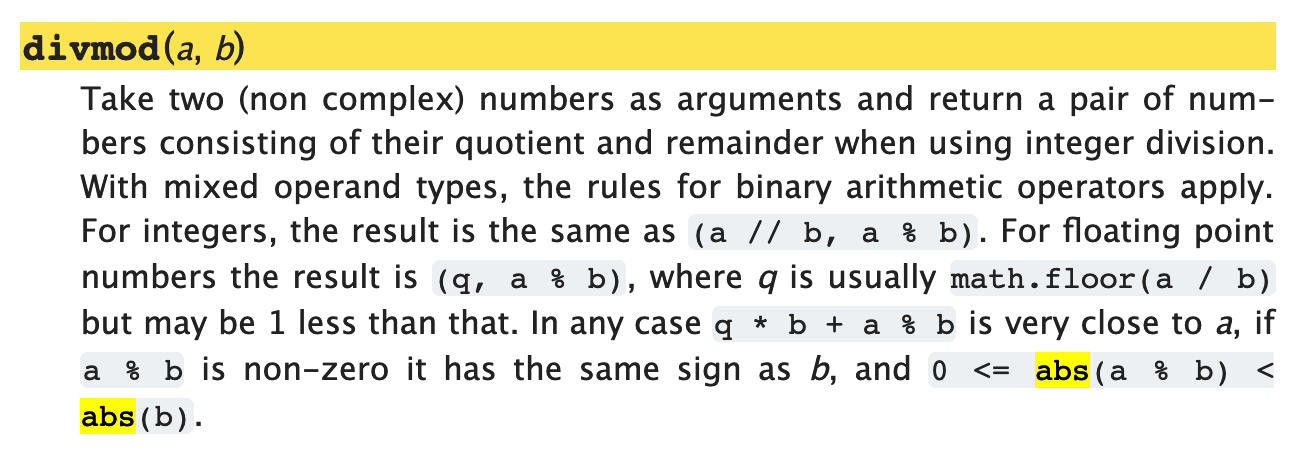• 当你调用这个函数的时候，括号里写的第一个参数，是被除数，第二个参数是除数 —— 此为该函数的输入；
• 而它的返回值，是一个元组（Tuple，至于这是什么东西，后面讲清楚），其中包括两个值，第一个是商，第二个是余 —— 此为该函数的输出。

print(divmod(11, 3))
a, b = divmod(11, 3)
print(a)
print(b)

print(divmod(3, 11))
a, b = divmod(3, 11)
print(a)
print(b)
(3, 2)
3
2
(0, 3)
0
3

可选位置参数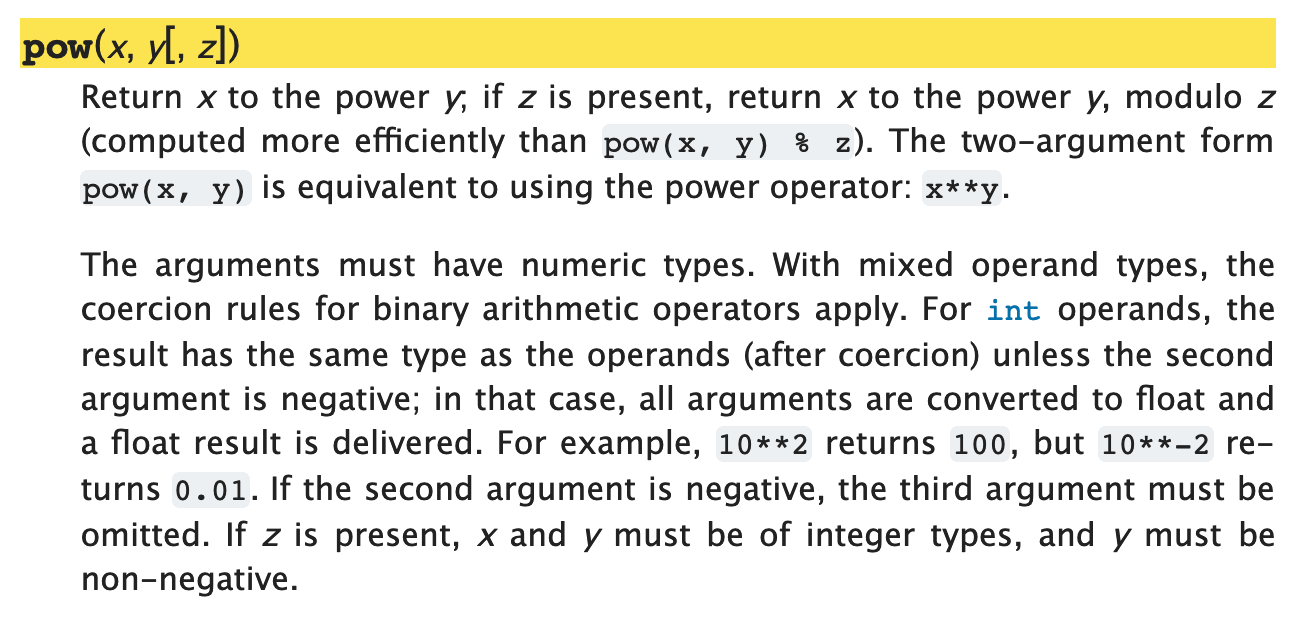• pow(x, y) —— 返回值是 x ** y
• pow(x, y, z) —— 返回值是 x ** y % z
print(pow(2, 3))
print(pow(2, 3, 4))
8
0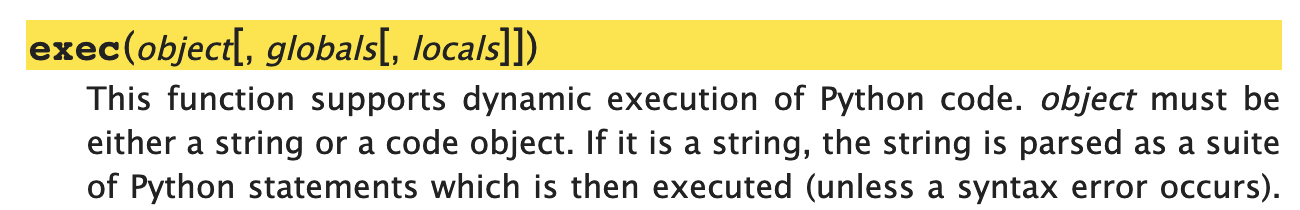• 没在方括号里的 object 是不可或缺的参数，调用时必须提供；
• 可以有第二个参数，第二个参数会被接收为 globals
• 在有第二个参数的情况下，第三个参数会被接收为 locals
• 但是，你没办法在不指定 globals 这个位置参数的情况下指定 locals……

可接收很多值的位置参数Class 也是函数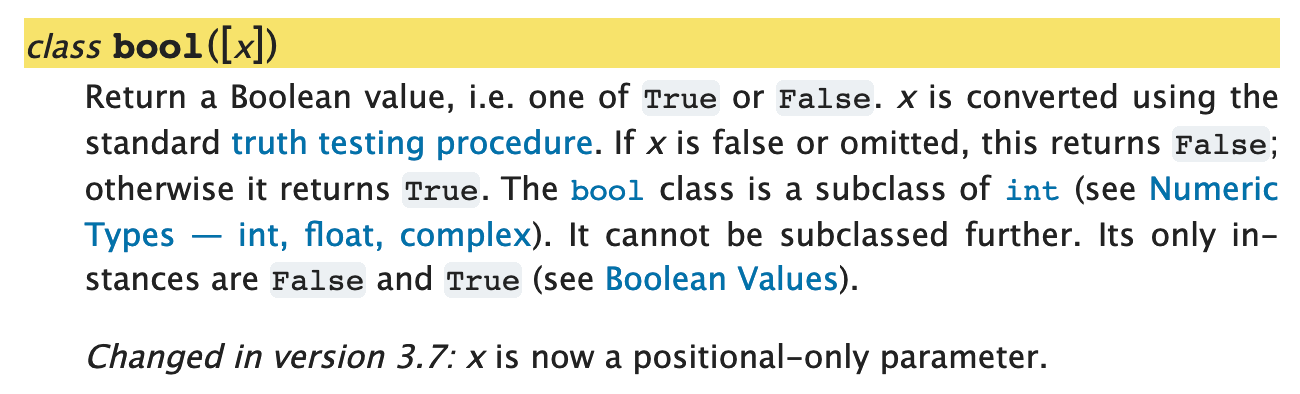print(bool())
print(bool(3.1415926))
print(bool(-3.1415926))
print(bool(1 == 2))
print(bool(None))
False
True
True
False
False

总结

• 你可以把函数当作一个产品，而你自己是这个产品的用户；
• 既然你是产品的用户，你要养成好习惯，一定要亲自阅读产品说明书；
• 调用函数的时候，注意可选位置参数的使用方法关键字参数的默认值
• 函数定义部分，注意两个符号就行了，[]=
• 所有的函数都有返回值，即便它内部不指定返回值，也有一个默认返回值：None
• 另外，一定要耐心阅读该函数在使用的时候需要注意什么 —— 产品说明书的主要作用就在这里……

1. ^ print() 函数的官方文档里，sep='' 肯定是 sep=' ' 的笔误 —— 可以用以下代码验证（写于 2019.02.14）：
print('a', 'b', sep='')
print('a', 'b')

2019.03.16, 有读者提醒：https://github.com/selfteaching/the-craft-of-selfteaching/issues/111